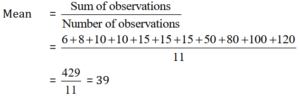# Data Handling Ncert Solution for Class 7 Maths Chapter 3 Exercise 3.2

Data Handling Class 7 Ex. 3.2;

New Ncert Solution for Class 7 Maths Chapter 3 Data Handling Free Solution;

Exercise 3.2

Question 1 :- The scores, in mathematics-test(out of 25)of 15-students, is as follows:
19, 25, 23, 20, 9, 20, 15, 10, 5, 16, 25, 20, 24, 12, 20
Find, the mode and median of this data. Are they same?

Solution 1:-
Given scores-data:
19, 25, 23, 20, 9, 20, 15, 10, 5, 16, 25, 20, 24, 12, 20
Taking arrangement of the given data in increasing order
5, 9, 10, 12, 15, 16 , 19, 20, 20, 20, 20, 23, 24, 25, 25

The-mode (a set of observations), is the observation that occurs, most-often.
Since, 20 occurs 4-times (highest)
So, Mode = 20
n = 15 (odd)    [ where, n = number of observation.]

the median, gives us the middle observation.

∴ Median = (n+1)/2 th term = (15+1)/2 = 8th term = 20
So, median = 20 and mode = 20
Finally, Mode and median are same as 20.

Question 2 :- The runs, scored in a cricket-match by 11-players is as follows:
6, 15, 120, 50, 100, 80, 10, 15, 8, 10, 15
Find the mean, mode and median of this data. Are the three same?

Solution 2:-
Given data:
6, 15, 120, 50, 100, 80, 10, 15, 8, 10, 15

Taking arrangment of the given data in increasing order, we get

6, 8, 10, 10, 15, 15, 15, 50, 80, 100, 120,Here, 15 occurs 3 times (highest)
∴ Mode = 15
n = 11 (odd)
∴ Median = (11+1)/2 th term = 6th term = 15
Thus mean = 39, mode = 15 and median = 15

No, the mean, mode and median are not given the same data.

Question 3 :- The weights, (in kg) of 15-students of a class, are:
38, 42, 35, 37, 45, 50, 32, 43, 43, 40, 36, 38, 43, 38, 47
(i) Find the mode and median of this data ?
(ii) Is there more than one mode?
Solution 3:-
Given data: 38, 42, 35, 37, 45, 50, 32, 43, 43, 40, 36, 38, 43, 38, 47

Arranging in increasing order, we get
32, 35, 36, 37, 38, 38, 38, 40, 42, 43, 43, 43, 45, 47, 50,

(i) Here, 38 and 43 occur 3 times (highest)
Thus mode = 38 and 43
Since, n = 15(odd)  [where, n = number of observation data.]
Median = (n+1)/2 th term =(15+1)/2 th term = 8th term = 40
Thus mode 38 and 43 and median = 40

(ii) Yes, the given data has two modes i.e. 38 and 43.

Question 4 :- Find the mode and median of the data:
13, 16, 12, 14, 19, 12, 14, 13, 14
Solution 4:-
13, 16, 12, 14, 19, 12, 14, 13, 14
Arranging the given data in increasing order, we get

12, 12, 13, 13, 14, 14, 14, 16, 19

Here, 14 occur 3 times (highest)
Thus, mode = 14
n = 9(odd)      [where, n = number of observation.]
∴ Median = (n+1)/2 th  term = (9+1)/2 th  term = 5th term = 14
Hence, mode = 14 and median = 14.

Question 5 :- Tell whether the statement is true or false.
(i) The mode is always one of the number in a data.
(ii) The mean is one of the numbers in a data.
(iii) The median is always one of the numbers in a data.
(iv) The data 6, 4, 3, 8, 9, 12, 13, 9 has mean 9.

Solution 5:-
(i) True
(ii) False
(iii) True
(iv) False.